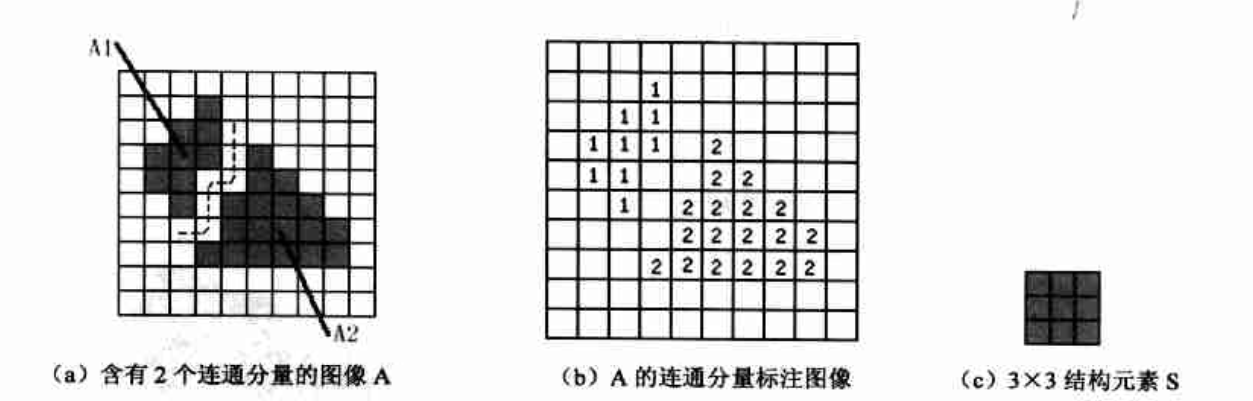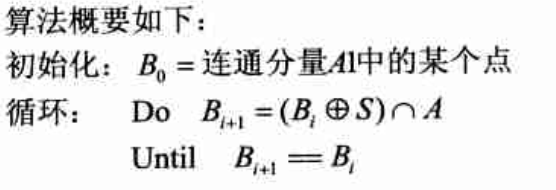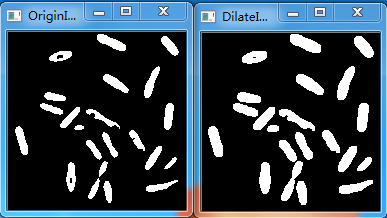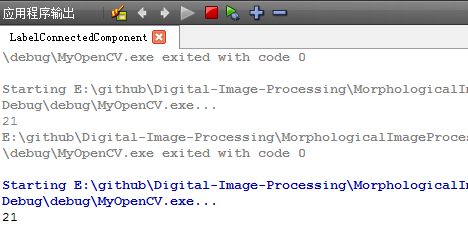2015-11-04 22:38:34 yuejuan0207 阅读数 11138
• ###### 图解Java数据结构和算法

1.算法是程序的灵魂，优秀的程序在对海量数据处理时，依然保持高速计算，就需要高效的数据结构和算法支撑。2.网上数据结构和算法的课程不少，但存在两个问题： 1)授课方式单一，大多是照着代码念一遍，数据结构和算法本身就比较难理解，对基础好的学员来说，还好一点，对基础不好的学生来说，基本上就是听天书了 2)说是讲数据结构和算法，但大多是挂羊头卖狗肉，算法讲的很少。 本课程针对上述问题，有针对性的进行了升级  3)授课方式采用图解+算法游戏的方式，让课程生动有趣好理解 4)系统全面的讲解了数据结构和算法, 除常用数据结构和算法外，还包括程序员常用10大算法：二分查找算法（非递归）、分治算法、动态规划算法、KMP算法、贪心算法、普里姆算法、克鲁斯卡尔算法、迪杰斯特拉算法、弗洛伊德算法、马踏棋盘算法。可以解决面试遇到的最短路径、最小生成树、最小连通图、动态规划等问题及衍生出的面试题，让你秒杀其他面试小伙伴 3.如果你不想永远都是代码工人，就需要花时间来研究下数据结构和算法。教程内容： 本教程是使用Java来讲解数据结构和算法，考虑到数据结构和算法较难，授课采用图解加算法游戏的方式。内容包括: 稀疏数组、单向队列、环形队列、单向链表、双向链表、环形链表、约瑟夫问题、栈、前缀、中缀、后缀表达式、中缀表达式转换为后缀表达式、递归与回溯、迷宫问题、八皇后问题、算法的时间复杂度、冒泡排序、选择排序、插入排序、快速排序、归并排序、希尔排序、基数排序(桶排序)、堆排序、排序速度分析、二分查找、插值查找、斐波那契查找、散列、哈希表、二叉树、二叉树与数组转换、二叉排序树(BST)、AVL树、线索二叉树、赫夫曼树、赫夫曼编码、多路查找树(B树B+树和B*树)、图、图的DFS算法和BFS、程序员常用10大算法、二分查找算法(非递归)、分治算法、动态规划算法、KMP算法、贪心算法、普里姆算法、克鲁斯卡尔算法、迪杰斯特拉算法、弗洛伊德算法马踏棋盘算法。 学习目标：通过学习，学员能掌握主流数据结构和算法的实现机制，开阔编程思路，提高优化程序的能力。

4187 人正在学习 去看看 佟刚

2、实验步骤

①读入图片，高斯滤波，再采用大律法选取阈值二值化图像。

②将二值图像进行反转（白色变黑色，黑色变白色），进行腐蚀操作（扩大气管与肺实质间距离，便于后面连通标记不会讲气管与肺实质粘连在一            块），进行区域连通标记，选取面积阈值，得到初始肺区域。

③提取初始肺区域边界，凸包算法进行凸包查找，计算相邻凸包点距离和周长比值，选定阈值，确定要修补的区域。对于靠近心脏附近的肺凹陷，            通过向量法查找凸点，计算相邻凸点距离和周长比值，选定阈值，确定要修补的区域。

④将修补后的轮廓生成掩膜，与原图像进行相乘得到修补后的肺实质区域。
3
、实验代码

K=gasssmooth(I);%高斯滤波平滑

[vm,vn]=size(K);

QW=zeros(vm,vn);

level = graythresh(K);%大律法阈值选取

BW =im2bw(K,level); %阈值二值化图像

p=imcomplement(BW); %图像二值反转

se=strel('square',2); %形态学腐蚀和膨胀操作用的模板，2*2矩形

se1=strel('disk',2);%形态学腐蚀和膨胀操作用的模板，半径为2圆形

bw1=imerode(p,se);%形态学腐蚀操作，扩大气管与肺实质间距离，便于连通标记时两者不粘连在一起，使得无法去除气管

[L,N]=bwlabel(bw1,4);%连通标记

s = regionprops(L,'Area');%将连通标记结果对应连通区域计算面积

bw2=ismember(L,find([s.Area]>=7000 &[s.Area]<=40000  ));%去除面积过大的（为背景）和面积过小的（为气管），得到肺实质初始区域

bw2=imdilate(bw2,se);  %进行形态学膨胀操作，需多次进行膨胀腐蚀操作，平滑标记后的区域。

bw3=imerode(bw2,se);%进行形态学腐蚀操作

bw2=imdilate(bw2,se);

bw3=imfill(bw3,'holes');%填充肺实质初始区域内部的孔洞，便于后面提取轮廓

[B,A]=bwboundaries(bw3);%边界提取

figure;imshow(QW)     %将修补后的边界显示在与原始图尺寸相同的图像，背景为黑色。

q=get(gca,'position');   %获得当前窗口位置，该处代码起至以下三行代码是为了获得与原始图像尺寸相同的图像。

q(1)=0;%设置左边距离值为零

q(2)=0;%设置右边距离值为零

set(gca,'position',q);

for g = 1:length(B)    %两段边界

hold on;

boundary = B{g};

qt=boundary;

m=length(boundary(:,1))

y=boundary(:,1);

x=boundary(:,2);

k=convhull(x,y);%凸包算法

n=length(k);

I=[x(k) y(k)];

h=length(I);

[p,t]=c_dis_neibor(k,I,m) ;   %计算凸包相邻凸点间的距离和周长比值。

index=find(t>=1 & t<9.46 );%选取比值阈值，确定需要修补的边界段，以下是对边界进行修补，包括靠近胸膜和靠近心脏两部分凹陷的修补

q=p(index-1);

w=p(index);

xx=zeros(1,1);

qq=zeros(1,1);

mm=zeros(1,1);

ww=zeros(1,1);

for j=1:length(w)  %凸包凸点修补，针对整个肺区域

st=q(j);

et=w(j);

if((et-st)<4)

sh=0;

elseif((et-st)<50)

ss=st+1;

ee=et-1;

sh=[ss:ee];

sh=sh(:);

else

ss=st+1;

ee=et-1;

hh=[ss:ee];

hh=hh(:);

nn=length(hh); %靠近心脏部分凹陷修补

for i=1:nn

q=hh(i);

if (i==1 )

v1=qt(hh(nn),:)-qt(hh(1),:);

v2=qt(hh(2),:)-qt(hh(1),:);

elseif (i==nn)

v1=qt(hh(nn-1),:)-qt(hh(nn),:);

v2=qt(hh(1),:)-qt(hh(nn),:);

else

v1=qt(hh(i-1),:)-qt(hh(i),:);

v2=qt(hh(i+1),:)-qt(hh(i),:);

end

r=det([v1;v2]);  %计算相邻两点间的向量叉乘a×b=x1*y2-x2*y1

if r>0        %大于零则为凸点，并将对应的点保存，用于后面计算相邻两凸点间比值

qq=[qq;q];

elseif r<0    %小于零则为凹点

mm=[mm;q];

end

end

qq=qq(find(qq));

bq=boundary(qq,:);

[pp,tt]=neibu_dis_neibor(qq,bq);%计算相邻两凸点间比值

index=find(tt>=1 &tt<=10.0 );  %选取阈值，确定要修补的边界段

pq=qq(index-1);

wq=qq(index);

for f=1:length(wq)   %将要修补的点保存，用于后面在原边界中去除这些点，进行修补

qs=pq(f)+1;

we=wq(f)-1;

rh=[qs:we];

rh=rh(:);

ww=[rh;ww];

end

ww=ww(find(ww));

sh=ww;

end

xx=[xx;sh];              %得到所有需要修补的点，

end

xx=xx(find(xx));

xx=xx';

qt(xx,:)=[];  %在原边界中去除要修补的点

plot(qt(:,2),qt(:,1),'W','LineWidth',1);%去除点后进行画线操作，形成新的边界轮廓，即为修补后的轮廓

end

frame=getframe(gcf,[0,0,vn,vm]); %获取当前窗口，并选取窗口范围[0,0,vn,vm]

im=frame2im(frame); %将获得的窗口生成图片

imwrite(im,'kk.jpg','jpg');%保存图片

生成的图片  kk.jpg  像素大小为512*512

clear

clc

I=rgb2gray(I);

[m,n]=size(I);

M=rgb2gray(M);

[q,k]=size(M);

IK=double(I); %进行图像操作时最好先转换为double型数据

MK=double(M);

J=MK(:,:,1)>210;  %将边界图像中灰度值大于210的点值置为1.

[L,num]=bwlabel(J,4);  %连通标记

F=L>1; %去除边界周围区域，

KK=imfill(F,'holes');  %填充边界内部

IK=uint8(IK);   %先将图片进行uint8数据转换，使两者类型一致才能进行后续相乘操作，否则会报错

KK=uint8(KK);

G=IK.*KK; %掩膜和原图相乘

imwrite(G,'xiubu22.jpg','jpg');

figure;

subplot(121);imshow(I);

subplot(122);imshow(G);

 生成最终结果   有问题欢迎探讨，如果有更好解决方法请联系我  1136958892

(8.07 KB, 下载次数: 0)

(10.36 KB, 下载次数: 0)

(146.93 KB, 下载次数: 0)

2019-09-01 17:11:33 webzhuce 阅读数 543
• ###### 图解Java数据结构和算法

1.算法是程序的灵魂，优秀的程序在对海量数据处理时，依然保持高速计算，就需要高效的数据结构和算法支撑。2.网上数据结构和算法的课程不少，但存在两个问题： 1)授课方式单一，大多是照着代码念一遍，数据结构和算法本身就比较难理解，对基础好的学员来说，还好一点，对基础不好的学生来说，基本上就是听天书了 2)说是讲数据结构和算法，但大多是挂羊头卖狗肉，算法讲的很少。 本课程针对上述问题，有针对性的进行了升级  3)授课方式采用图解+算法游戏的方式，让课程生动有趣好理解 4)系统全面的讲解了数据结构和算法, 除常用数据结构和算法外，还包括程序员常用10大算法：二分查找算法（非递归）、分治算法、动态规划算法、KMP算法、贪心算法、普里姆算法、克鲁斯卡尔算法、迪杰斯特拉算法、弗洛伊德算法、马踏棋盘算法。可以解决面试遇到的最短路径、最小生成树、最小连通图、动态规划等问题及衍生出的面试题，让你秒杀其他面试小伙伴 3.如果你不想永远都是代码工人，就需要花时间来研究下数据结构和算法。教程内容： 本教程是使用Java来讲解数据结构和算法，考虑到数据结构和算法较难，授课采用图解加算法游戏的方式。内容包括: 稀疏数组、单向队列、环形队列、单向链表、双向链表、环形链表、约瑟夫问题、栈、前缀、中缀、后缀表达式、中缀表达式转换为后缀表达式、递归与回溯、迷宫问题、八皇后问题、算法的时间复杂度、冒泡排序、选择排序、插入排序、快速排序、归并排序、希尔排序、基数排序(桶排序)、堆排序、排序速度分析、二分查找、插值查找、斐波那契查找、散列、哈希表、二叉树、二叉树与数组转换、二叉排序树(BST)、AVL树、线索二叉树、赫夫曼树、赫夫曼编码、多路查找树(B树B+树和B*树)、图、图的DFS算法和BFS、程序员常用10大算法、二分查找算法(非递归)、分治算法、动态规划算法、KMP算法、贪心算法、普里姆算法、克鲁斯卡尔算法、迪杰斯特拉算法、弗洛伊德算法马踏棋盘算法。 学习目标：通过学习，学员能掌握主流数据结构和算法的实现机制，开阔编程思路，提高优化程序的能力。

4187 人正在学习 去看看 佟刚

# 基本概念

提取连通分量的过程实际上也是标注连通分量的过程，通常的做法是给原图像中的每个连通区分配一个唯一代表该区域的编号，在输出图像中该连通区内的所有的像素值就赋值为该区域的编号，我们将这样的输出图像称为标注图像。这里介绍一种基于形态学的膨胀操作的提取连通分量的方法。# 示例演示

我们用OpenCV实现连通分量提取函数，然后进行细菌计数。完整工程代码。

``````/*
*only process binary image
*iseight = true means eight connected, otherwise four connected
*/
int LabelConnectedComponent(const cv::Mat &src, cv::Mat &dst, bool iseight = true)
{
Mat structelement;
if(iseight)
structelement = getStructuringElement(MORPH_RECT, Size(3, 3));
else
structelement = getStructuringElement(MORPH_CROSS, Size(3, 3));

dst = Mat::ones(src.size(), src.type());
Mat tmp = Mat::ones(src.size(), src.type()); // save last reuslt image
Mat img = Mat::ones(src.size(), src.type()); //image B
int labelnum = 0; //label of connected component

Mat backupsrc;
src.copyTo(backupsrc);
for(int i = 0; i < backupsrc.rows; i++)
{
for(int j = 0; j < backupsrc.cols; j++)
{
if(backupsrc.at<uchar>(i, j) == 255)
{
Mat img = Mat::ones(src.size(), src.type());
img.at<uchar>(i, j) = 255;
img.copyTo(tmp);  //Temporary save
labelnum++;

while(true)  // until not change
{
cv::dilate(img, img, structelement);
bitwise_and(img, src, img);
//if img do not change, this connected component is finished
if (cv::countNonZero(img - tmp) == 0)
break;
img.copyTo(tmp);
}

//label the connected component
for(int r = 0; r < img.rows; r++)
{
for(int c = 0; c < img.cols; c++)
{
if(img.at<uchar>(r, c) == 255)
{
backupsrc.at<uchar>(r, c) = 0;
dst.at<uchar>(r, c) = labelnum;
}
}
}
}
}
}
return labelnum;
}
``````

先对原始图像进行膨胀然后进行细菌统计，实际的细菌个数为21。2015-05-05 21:22:32 bblr001 阅读数 2411
• ###### 图解Java数据结构和算法

1.算法是程序的灵魂，优秀的程序在对海量数据处理时，依然保持高速计算，就需要高效的数据结构和算法支撑。2.网上数据结构和算法的课程不少，但存在两个问题： 1)授课方式单一，大多是照着代码念一遍，数据结构和算法本身就比较难理解，对基础好的学员来说，还好一点，对基础不好的学生来说，基本上就是听天书了 2)说是讲数据结构和算法，但大多是挂羊头卖狗肉，算法讲的很少。 本课程针对上述问题，有针对性的进行了升级  3)授课方式采用图解+算法游戏的方式，让课程生动有趣好理解 4)系统全面的讲解了数据结构和算法, 除常用数据结构和算法外，还包括程序员常用10大算法：二分查找算法（非递归）、分治算法、动态规划算法、KMP算法、贪心算法、普里姆算法、克鲁斯卡尔算法、迪杰斯特拉算法、弗洛伊德算法、马踏棋盘算法。可以解决面试遇到的最短路径、最小生成树、最小连通图、动态规划等问题及衍生出的面试题，让你秒杀其他面试小伙伴 3.如果你不想永远都是代码工人，就需要花时间来研究下数据结构和算法。教程内容： 本教程是使用Java来讲解数据结构和算法，考虑到数据结构和算法较难，授课采用图解加算法游戏的方式。内容包括: 稀疏数组、单向队列、环形队列、单向链表、双向链表、环形链表、约瑟夫问题、栈、前缀、中缀、后缀表达式、中缀表达式转换为后缀表达式、递归与回溯、迷宫问题、八皇后问题、算法的时间复杂度、冒泡排序、选择排序、插入排序、快速排序、归并排序、希尔排序、基数排序(桶排序)、堆排序、排序速度分析、二分查找、插值查找、斐波那契查找、散列、哈希表、二叉树、二叉树与数组转换、二叉排序树(BST)、AVL树、线索二叉树、赫夫曼树、赫夫曼编码、多路查找树(B树B+树和B*树)、图、图的DFS算法和BFS、程序员常用10大算法、二分查找算法(非递归)、分治算法、动态规划算法、KMP算法、贪心算法、普里姆算法、克鲁斯卡尔算法、迪杰斯特拉算法、弗洛伊德算法马踏棋盘算法。 学习目标：通过学习，学员能掌握主流数据结构和算法的实现机制，开阔编程思路，提高优化程序的能力。

4187 人正在学习 去看看 佟刚

```#include <iostream>
#include "cv.h"
#include "cxcore.h"
#include "highgui.h"
#include <vector>

#pragma comment(lib, "cv")
#pragma comment(lib, "cxcore")
#pragma comment(lib, "highgui")

using namespace std;

static IplImage* MarkImage;
static CvPoint* PointArray ;
void CreateBuff(int width, int height)
{
int y;
MarkImage = cvCreateImage(cvSize(width, height), IPL_DEPTH_8U, 1);
PointArray = (CvPoint*)malloc(width*height*sizeof(CvPoint));

cvZero(MarkImage);
memset(PointArray, 0, width*height*sizeof(CvPoint));
}

void ReleaseBuff()
{
cvReleaseImage(&MarkImage);
free(PointArray);
}

typedef struct Region
{
int size;
CvRect rect;
}Region;

void FilterMaxHeightWidthRegionForRect(IplImage* srcImage, vector<Region>& Reg)
{
int hIndex = 0, wIndex = 0, Position = 0;
int iStartNum = 0, iEndNum = 0, EdgeNum = 0;
int XValue = 0, YValue = 0, dIndex = 0;

int width = srcImage->width;
int height= srcImage->height;

int MinXValue = 0, MaxXValue = 0, MinYValue = 0, MaxYValue = 0;
int PointArrayCnt;
for (hIndex = 0; hIndex < height; hIndex++)
{
for (wIndex = 0; wIndex < width; wIndex++)
{
Position = hIndex*srcImage->widthStep + wIndex;

if (srcImage->imageData[Position] == 0 )
continue;

if (MarkImage->imageData[Position] != 0)
continue;

iStartNum = -1; iEndNum = 0;  EdgeNum = 0;
PointArrayCnt = 0;
PointArray[PointArrayCnt] = cvPoint(wIndex, hIndex);

MarkImage->imageData[Position] = 128;

MinXValue = MaxXValue = wIndex;
MinYValue = MaxYValue = hIndex;

while(iStartNum < iEndNum)
{
iStartNum = iStartNum + 1;

XValue = PointArray[iStartNum].x;
YValue = PointArray[iStartNum].y;

MinXValue = MinXValue < XValue ? MinXValue : XValue;
MaxXValue = MaxXValue > XValue ? MaxXValue : XValue;
MinYValue = MinYValue < YValue ? MinYValue : YValue;
MaxYValue = MaxYValue > YValue ? MaxYValue : YValue;

if(XValue < 0 || XValue >= width
|| YValue < 0 || YValue >= height)
continue;

Position = YValue*srcImage->widthStep + XValue;

if (XValue - 1 >= 0 && YValue - 1 >= 0
&& MarkImage->imageData[Position - srcImage->widthStep - 1] == 0
&& srcImage->imageData[Position - srcImage->widthStep - 1] != 0)
{

++PointArrayCnt;
PointArray[PointArrayCnt] = cvPoint(XValue - 1, YValue - 1);
MarkImage->imageData[Position - srcImage->widthStep - 1] = 128;
}

if (XValue >= 0 && YValue - 1 >= 0
&& MarkImage->imageData[Position - srcImage->widthStep] == 0
&& srcImage->imageData[Position - srcImage->widthStep] != 0)
{

++PointArrayCnt;
PointArray[PointArrayCnt] = cvPoint(XValue, YValue - 1);
MarkImage->imageData[Position - srcImage->widthStep] = 128;
}

if (XValue + 1 < width && YValue - 1 >= 0
&& MarkImage->imageData[Position - srcImage->widthStep + 1] == 0
&& srcImage->imageData[Position - srcImage->widthStep + 1] != 0)
{

++PointArrayCnt;
PointArray[PointArrayCnt] = cvPoint(XValue + 1, YValue - 1);
MarkImage->imageData[Position - srcImage->widthStep + 1] = 128;
}

if (XValue + 1 < width && YValue >= 0
&& MarkImage->imageData[Position + 1] == 0
&& srcImage->imageData[Position + 1] != 0)
{

++PointArrayCnt;
PointArray[PointArrayCnt] = cvPoint(XValue + 1, YValue);
MarkImage->imageData[Position + 1] = 128;
}

if (XValue + 1 < width && YValue + 1 < height
&& MarkImage->imageData[Position + srcImage->widthStep + 1] == 0
&& srcImage->imageData[Position + srcImage->widthStep + 1] != 0)
{

++PointArrayCnt;
PointArray[PointArrayCnt] = cvPoint(XValue + 1, YValue + 1);
MarkImage->imageData[Position + srcImage->widthStep + 1] = 128;
}

if (XValue >= 0 && YValue + 1 < height
&& MarkImage->imageData[Position + srcImage->widthStep] == 0
&& srcImage->imageData[Position + srcImage->widthStep] != 0)
{

++PointArrayCnt;
PointArray[PointArrayCnt] = cvPoint(XValue, YValue + 1);
MarkImage->imageData[Position + srcImage->widthStep] = 128;
}

if (XValue - 1 >= 0 && YValue + 1 < height
&& MarkImage->imageData[Position + srcImage->widthStep - 1] == 0
&& srcImage->imageData[Position + srcImage->widthStep - 1] != 0)
{

++PointArrayCnt;
PointArray[PointArrayCnt] = cvPoint(XValue - 1, YValue + 1);
MarkImage->imageData[Position + srcImage->widthStep - 1] = 128;
}

if (XValue - 1 >= 0 && YValue >= 0
&&MarkImage->imageData[Position - 1] == 0
&& srcImage->imageData[Position - 1] != 0)
{

++PointArrayCnt;
PointArray[PointArrayCnt] = cvPoint(XValue - 1, YValue);
MarkImage->imageData[Position - 1] = 128;
}

iEndNum = PointArrayCnt;
}
if(PointArrayCnt >0)
{
Region tmpRegion;
tmpRegion.size = PointArrayCnt;
tmpRegion.rect.x = MinXValue;
tmpRegion.rect.y = MinYValue;
tmpRegion.rect.width = MaxXValue - MinXValue + 1;
tmpRegion.rect.height = MaxYValue - MinYValue + 1;

Reg.push_back(tmpRegion);
memset(PointArray, 0, width*height*sizeof(CvPoint));
}
}
}
}
void main()
{
int width = img->width;
int height= img->height;

IplImage* tmp = cvCreateImage(cvSize(width, height), IPL_DEPTH_8U, 1);
cvZero(tmp);
cvThreshold(img, tmp, 160, 255, CV_THRESH_BINARY);
cvNamedWindow("tmp");
cvShowImage("tmp", tmp);
CreateBuff(width, height);

vector<Region> region;
FilterMaxHeightWidthRegionForRect(tmp, region);
cout<<region.size()<<endl;
cvNamedWindow("Draw");
for(int i=0; i<region.size(); ++i)
{
CvPoint pt1, pt2;
pt1.x = region.at(i).rect.x-1;
pt1.y = region.at(i).rect.y-1;
pt2.x = pt1.x + region.at(i).rect.width+1;
pt2.y = pt1.y + region.at(i).rect.height+1;

cvRectangle(tmp, pt1, pt2, CV_RGB(255,255,255), 1, 8, 0);

cout<<"size = "<<region.at(i).size<<endl;
}
cvShowImage("Draw", tmp);
cvWaitKey(0);

system("pause");
}

```

//

//2017-08-03 09:42:36 chaihuimin 阅读数 2265
• ###### 图解Java数据结构和算法

1.算法是程序的灵魂，优秀的程序在对海量数据处理时，依然保持高速计算，就需要高效的数据结构和算法支撑。2.网上数据结构和算法的课程不少，但存在两个问题： 1)授课方式单一，大多是照着代码念一遍，数据结构和算法本身就比较难理解，对基础好的学员来说，还好一点，对基础不好的学生来说，基本上就是听天书了 2)说是讲数据结构和算法，但大多是挂羊头卖狗肉，算法讲的很少。 本课程针对上述问题，有针对性的进行了升级  3)授课方式采用图解+算法游戏的方式，让课程生动有趣好理解 4)系统全面的讲解了数据结构和算法, 除常用数据结构和算法外，还包括程序员常用10大算法：二分查找算法（非递归）、分治算法、动态规划算法、KMP算法、贪心算法、普里姆算法、克鲁斯卡尔算法、迪杰斯特拉算法、弗洛伊德算法、马踏棋盘算法。可以解决面试遇到的最短路径、最小生成树、最小连通图、动态规划等问题及衍生出的面试题，让你秒杀其他面试小伙伴 3.如果你不想永远都是代码工人，就需要花时间来研究下数据结构和算法。教程内容： 本教程是使用Java来讲解数据结构和算法，考虑到数据结构和算法较难，授课采用图解加算法游戏的方式。内容包括: 稀疏数组、单向队列、环形队列、单向链表、双向链表、环形链表、约瑟夫问题、栈、前缀、中缀、后缀表达式、中缀表达式转换为后缀表达式、递归与回溯、迷宫问题、八皇后问题、算法的时间复杂度、冒泡排序、选择排序、插入排序、快速排序、归并排序、希尔排序、基数排序(桶排序)、堆排序、排序速度分析、二分查找、插值查找、斐波那契查找、散列、哈希表、二叉树、二叉树与数组转换、二叉排序树(BST)、AVL树、线索二叉树、赫夫曼树、赫夫曼编码、多路查找树(B树B+树和B*树)、图、图的DFS算法和BFS、程序员常用10大算法、二分查找算法(非递归)、分治算法、动态规划算法、KMP算法、贪心算法、普里姆算法、克鲁斯卡尔算法、迪杰斯特拉算法、弗洛伊德算法马踏棋盘算法。 学习目标：通过学习，学员能掌握主流数据结构和算法的实现机制，开阔编程思路，提高优化程序的能力。

4187 人正在学习 去看看 佟刚

# 利用OpenCV求取图像多轮廓质心，并在输出图像上显示质心坐标

2015-01-07 20:27:06 u010278305 阅读数 11049
• ###### 图解Java数据结构和算法

1.算法是程序的灵魂，优秀的程序在对海量数据处理时，依然保持高速计算，就需要高效的数据结构和算法支撑。2.网上数据结构和算法的课程不少，但存在两个问题： 1)授课方式单一，大多是照着代码念一遍，数据结构和算法本身就比较难理解，对基础好的学员来说，还好一点，对基础不好的学生来说，基本上就是听天书了 2)说是讲数据结构和算法，但大多是挂羊头卖狗肉，算法讲的很少。 本课程针对上述问题，有针对性的进行了升级  3)授课方式采用图解+算法游戏的方式，让课程生动有趣好理解 4)系统全面的讲解了数据结构和算法, 除常用数据结构和算法外，还包括程序员常用10大算法：二分查找算法（非递归）、分治算法、动态规划算法、KMP算法、贪心算法、普里姆算法、克鲁斯卡尔算法、迪杰斯特拉算法、弗洛伊德算法、马踏棋盘算法。可以解决面试遇到的最短路径、最小生成树、最小连通图、动态规划等问题及衍生出的面试题，让你秒杀其他面试小伙伴 3.如果你不想永远都是代码工人，就需要花时间来研究下数据结构和算法。教程内容： 本教程是使用Java来讲解数据结构和算法，考虑到数据结构和算法较难，授课采用图解加算法游戏的方式。内容包括: 稀疏数组、单向队列、环形队列、单向链表、双向链表、环形链表、约瑟夫问题、栈、前缀、中缀、后缀表达式、中缀表达式转换为后缀表达式、递归与回溯、迷宫问题、八皇后问题、算法的时间复杂度、冒泡排序、选择排序、插入排序、快速排序、归并排序、希尔排序、基数排序(桶排序)、堆排序、排序速度分析、二分查找、插值查找、斐波那契查找、散列、哈希表、二叉树、二叉树与数组转换、二叉排序树(BST)、AVL树、线索二叉树、赫夫曼树、赫夫曼编码、多路查找树(B树B+树和B*树)、图、图的DFS算法和BFS、程序员常用10大算法、二分查找算法(非递归)、分治算法、动态规划算法、KMP算法、贪心算法、普里姆算法、克鲁斯卡尔算法、迪杰斯特拉算法、弗洛伊德算法马踏棋盘算法。 学习目标：通过学习，学员能掌握主流数据结构和算法的实现机制，开阔编程思路，提高优化程序的能力。

4187 人正在学习 去看看 佟刚

1、二值化

2、开操作

3、连通区域提取

4、连通区域的重心提取

5、BoundingBox提取

```%function:
%     获取感兴趣区域，并进行提取、标记
%     源图像位于E:\ProgramFiles\MATLAB\R2013b\toolbox\images\imdemos
%     具体路径因个人电脑而异
%date:2015-1-7
%author:chenyanan

%清空变量，读取图像，并显示其属性
clear;close all
whos,

%显示原始图像
figure('name','myapp'),
subplot(2,2,1),imshow(src),title('src')

%用ostu方法获取二值化阈值，进行二值化并进行显示
level=graythresh(src);
bw=im2bw(src,level);
subplot(2,2,2),imshow(bw),title('bw')

%运用开操作消去噪点
se = strel('disk',2);
openbw=imopen(bw,se);
subplot(2,2,3),imshow(openbw),title('open')

%获取连通区域，并进行显示
L = bwlabel(openbw,4);
RGB = label2rgb(L);
subplot(2,2,4),imshow(RGB),title('rgb')

%获取区域的'basic'属性， 'Area', 'Centroid', and 'BoundingBox'
stats = regionprops(openbw, 'basic');
centroids = cat(1, stats.Centroid);
figure('name','regionprops'),
%绘制开操作之后的二值化图像
imshow(openbw),title('centroids')
hold on
%绘制重心
plot(centroids(:,1), centroids(:,2), 'b*'),
%绘制感兴趣区域ROI
for i=1:size(stats)
rectangle('Position',[stats(i).BoundingBox],'LineWidth',2,'LineStyle','--','EdgeColor','r'),
end
hold off```